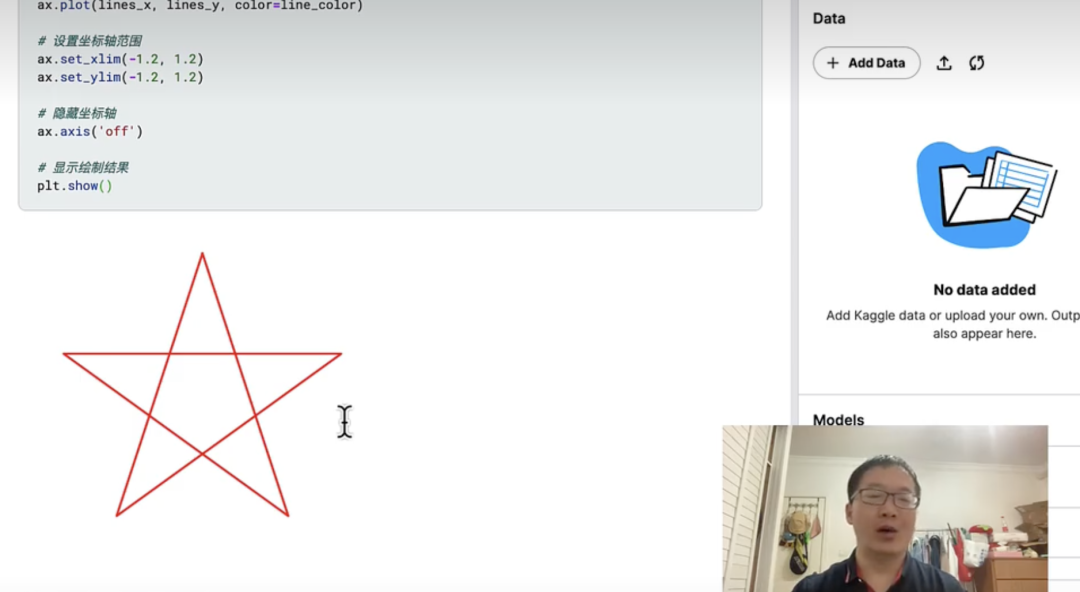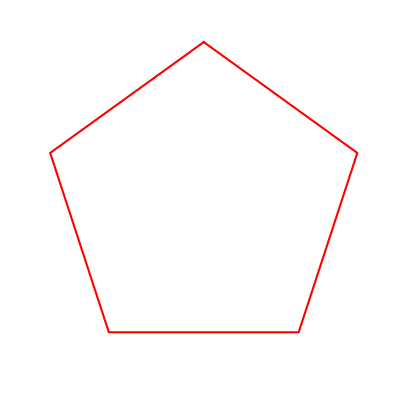Deleon 2023-05-16 67 0 0 0 0
ChatGPT,ChatGPT技巧,Prompt,范例演示：如何用ChatGPT绘制一个五角星？⭐️prompt=角色+任务+要求+提示【步骤拆解、范例说明，技巧点拨等】用大白话解释就是要告诉chatgpt：你是谁？要做什么？要做成什么样？要怎么做？…

# 范例演示：如何用ChatGPT绘制一个五角星？⭐️

prompt = 角色 + 任务 + 要求 + 提示【步骤拆解、范例说明，技巧点拨等】``from torchkeras.chatgpt import ChatGPT api_key = None#'sk-xx'chatgpt = ChatGPT(api_key=api_key,                  max_chat_rounds=3, #支持3轮上下文连续对话                  temperature=0                 )``
``Hello! How can I assist you today?register magic %%chatgpt sucessed ...``

## 一，任务

``%%chatgpt 任务：请用Python代码绘制一个五角星。``
``以下是绘制五角星的Python代码：```pythonimport turtle# 创建一个画布canvas = turtle.Screen()# 创建一个海龟对象pen = turtle.Turtle()# 设置画笔颜色和填充颜色pen.color("red", "yellow")# 开始填充pen.begin_fill()# 绘制五角星for i in range(5):    pen.forward(200)    pen.right(144)# 结束填充pen.end_fill()# 隐藏海龟pen.hideturtle()# 点击关闭窗口canvas.exitonclick()```运行代码后，会弹出一个窗口，里面绘制了一个红色边框、黄色填充的五角星。``

## 二，任务+要求

``%%chatgpt 任务：请用Python代码绘制一个五角星。要求：请使用matplotlib这个库，线条使用红色。``
``以下是使用matplotlib库绘制五角星的Python代码：```pythonimport matplotlib.pyplot as pltimport numpy as np# 创建一个画布fig, ax = plt.subplots()# 设置画布大小fig.set_size_inches(5, 5)# 设置线条颜色line_color = 'red'# 计算五角星的顶点坐标theta = np.linspace(0, 2*np.pi, 6)x = np.sin(theta)y = np.cos(theta)# 绘制五角星ax.plot(x, y, color=line_color)# 设置坐标轴范围ax.set_xlim(-1.2, 1.2)ax.set_ylim(-1.2, 1.2)# 隐藏坐标轴ax.axis('off')# 显示绘制结果plt.show()```运行代码后，会弹出一个窗口，里面绘制了一个红色线条的五角星。``## 三，角色+任务+要求

``%%chatgpt 角色：假设你是一个擅长绘图的Python程序员。任务：请用Python代码绘制一个五角星。要求：请使用matplotlib这个库，线条使用红色。``
``以下是使用matplotlib库绘制五角星的Python代码：```pythonimport matplotlib.pyplot as pltimport numpy as np# 创建一个画布fig, ax = plt.subplots()# 设置画布大小fig.set_size_inches(5, 5)# 设置线条颜色line_color = 'red'# 计算五角星的顶点坐标theta = np.linspace(0, 2*np.pi, 6)x = np.sin(theta)y = np.cos(theta)# 绘制五角星ax.plot(x, y, color=line_color)# 设置坐标轴范围ax.set_xlim(-1.2, 1.2)ax.set_ylim(-1.2, 1.2)# 隐藏坐标轴ax.axis('off')# 显示绘制结果plt.show()```运行代码后，会弹出一个窗口，里面绘制了一个红色线条的五角星。``## 四，角色+任务+要求+提示

``%%chatgpt 角色：假设你是一个有着丰富经验的Python程序员。任务：请用Python代码绘制一个五角星。要求：请使用matplotlib这个库，线条使用红色。提示：五角星需要先计算五个顶点，然后在每间隔一个顶点的两个顶点之间两两进行连线。``
``以下是使用matplotlib库绘制五角星的Python代码：```pythonimport matplotlib.pyplot as pltimport numpy as np# 创建一个画布fig, ax = plt.subplots()# 设置画布大小fig.set_size_inches(5, 5)# 设置线条颜色line_color = 'red'# 计算五角星的顶点坐标theta = np.linspace(0, 2*np.pi, 6)x = np.sin(theta)y = np.cos(theta)# 计算五角星的线段坐标lines_x = [x, x, x, x, x, x]lines_y = [y, y, y, y, y, y]# 绘制五角星ax.plot(lines_x, lines_y, color=line_color)# 设置坐标轴范围ax.set_xlim(-1.2, 1.2)ax.set_ylim(-1.2, 1.2)# 隐藏坐标轴ax.axis('off')# 显示绘制结果plt.show()```运行代码后，会弹出一个窗口，里面绘制了一个红色线条的五角星。``

Tag: ChatGPT ChatGPT技巧 Prompt

(一分鍾即可完成註冊!)